# Math > Year 2

## Making Patterns with Shapes

Pattern
A pattern is defined as a repeated arrangement or set of numbers, shapes, colours, and so on.

Example 1: Find the missing shape.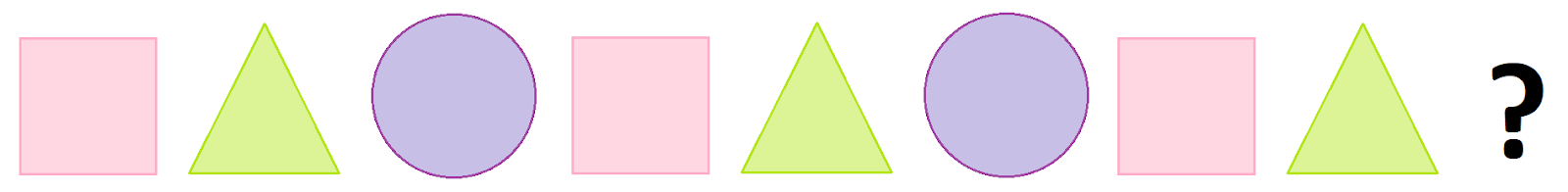Explanation:

The pattern is square, triangle, and circle. These three shapes are repeated continuously.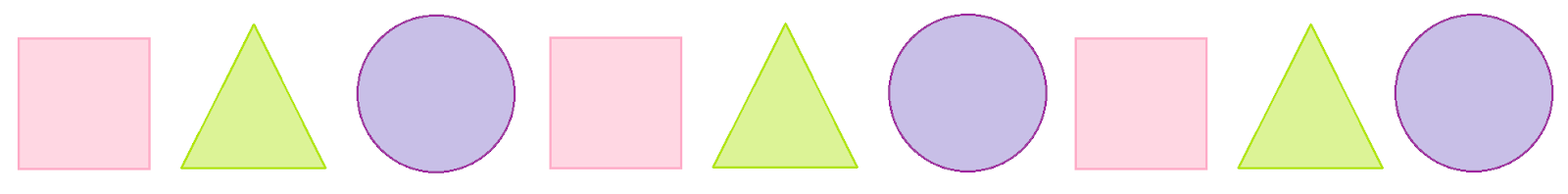Therefore, the missing shape is a circle.

Example 2: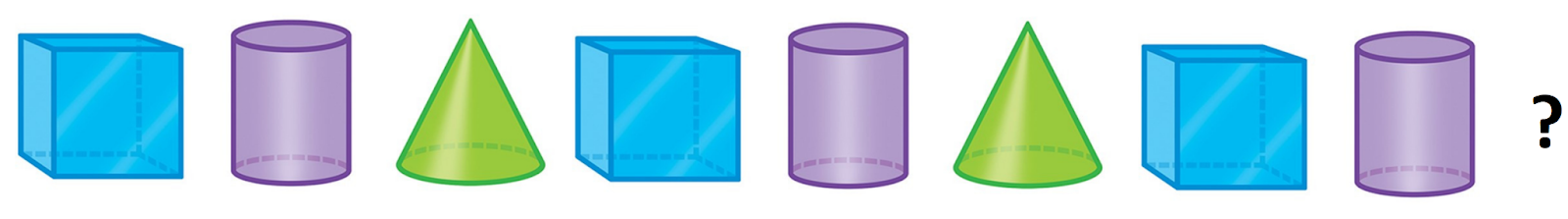Explanation:

Starting from the left, the pattern is a cube, cylinder, cone, cube, cylinder, cone, and so on.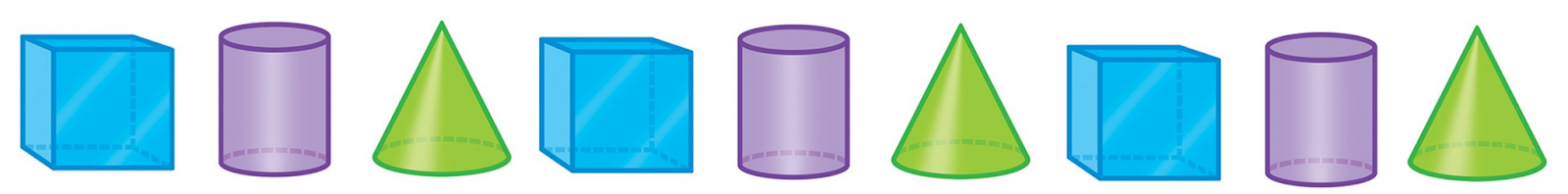The missing shape is a cone.

Some patterns can be made by turning the shapes in different ways.

We can turn the shapes in two ways: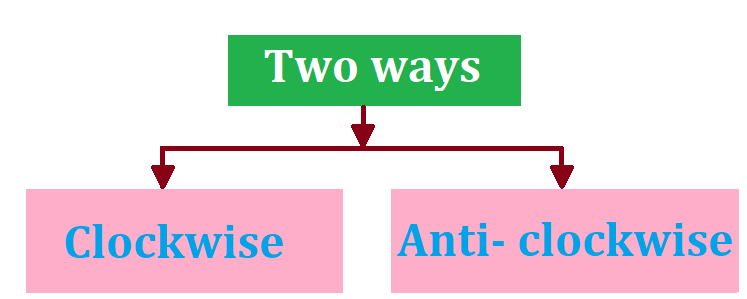Clockwise is defined as the direction when something turns in the same direction as the hands in a clock move.

Anti-clockwise is defined as the direction when something turns in the opposite direction to the hands in a clock.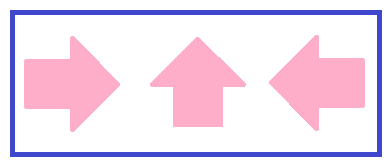The arrow has been moved each time in an anticlockwise direction.

Steps to find the rule in a pattern-

1. Observe the first shape and then compare it with the second shape.

2. Then we will figure out if it is moving in a clockwise direction or in an anticlockwise direction.

How to find the missing shape in a pattern?

Let’s learn with an example: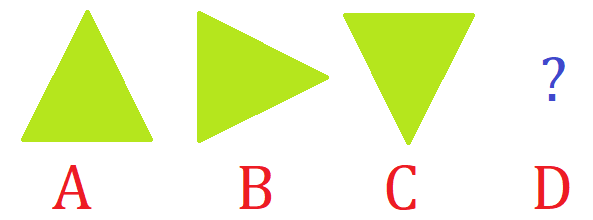1. Observe the first shape.

2. Compare with the second shape

3. In this, the triangle is turning a quarter-turn in the clockwise direction.

4. Turn the figure C quarter-turn in the clockwise direction to get the figure D.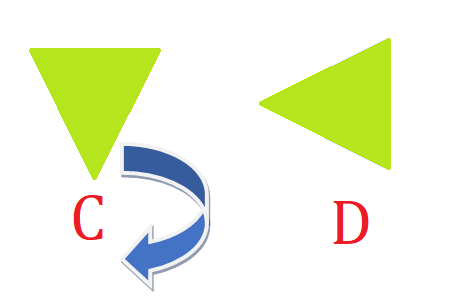Let’s try a question:

Question: The given shapes are making a pattern. The given shapes are moving in a ______ direction.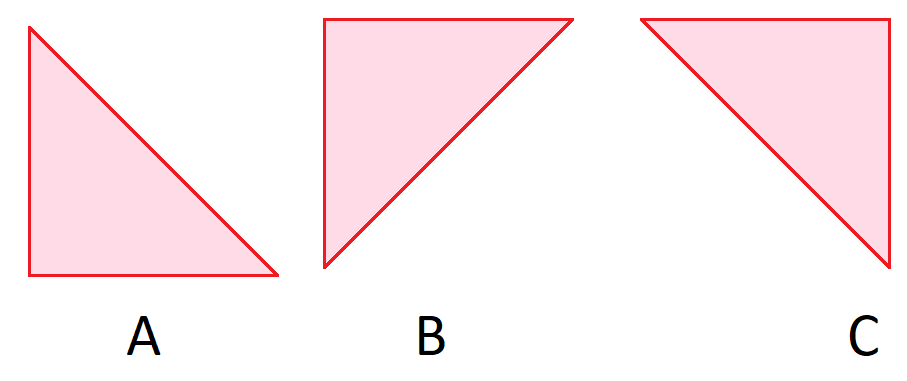Explanation:

The given shapes are moving in a clockwise direction.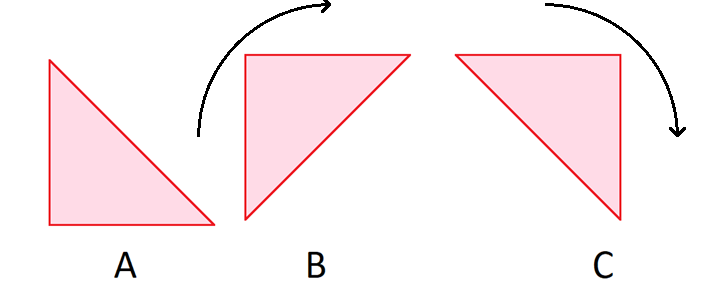//